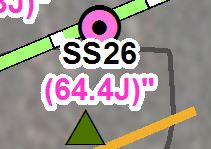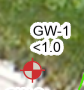# Label Expressions - Adding RGB Values to text

1491
3
03-05-2019 03:20 PMNew Contributor III

So, I get my label expression to color right with text formatting callouts (<CLR></CLR>),

BUT

There's a stupid double quote that winds up on the end of my label, below:I've tried deleting each quote in my VBscript, no dice.

My VB Script:

[SI_boring_previous.LOCATION_NAME] & vbnewline &"<CLR red = '255' green = '51' blue = '204'>"&"("&[gis_join\$.Pb_VALUE]&[gis_join\$.F4]&")""</CLR>"

Any thoughts?  bug?

Tags (1)
3 RepliesbyMVP Esteemed Contributor

This works, adding a & near the end fixed it

``[SI_boring_previous.LOCATION_NAME] & vbnewline & "<CLR red = '255' green = '51' blue = '204'>" & "(" &[gis_join\$.Pb_VALUE]&[gis_join\$.F4]&")" & "</CLR>"‍‍‍‍‍‍‍‍``

When the expressions get this complex, I like clicking the advanced button and using a function so you can use line continuations and see what you are doing:

``````Function FindLabel ( [SI_boring_previous.LOCATION_NAME],^
[gis_join\$.Pb_VALUE],^
[gis_join\$.F4] )
FindLabel = [SI_boring_previous.LOCATION_NAME] & vbnewline &^
"<CLR red = '255' green = '51' blue = '204'>" &^
"(" & [gis_join\$.Pb_VALUE] & [gis_join\$.F4] & ")" &^
"</CLR>"
End Function‍‍‍‍‍‍‍‍‍‍‍‍‍‍‍‍‍‍‍‍‍‍‍‍‍‍‍‍‍‍‍‍‍‍‍‍‍‍‍‍‍‍‍‍‍‍‍‍‍‍‍‍‍‍‍‍‍‍‍‍‍‍‍‍‍‍‍‍‍‍‍‍``````

Python is so much easier using its string formatting:

``````def FindLabel ( [SI_boring_previous.LOCATION_NAME],^
[gis_join\$.Pb_VALUE],^
[gis_join\$.F4]):
fmt =  "{}\r<CLR red='255' green='51' blue='204'>({}{})</CLR>"
return fmt.format(
[SI_boring_previous.LOCATION_NAME],
[gis_join\$.Pb_VALUE],
[gis_join\$.F4])
‍‍‍‍‍‍‍‍‍‍‍‍‍‍‍‍‍‍‍‍‍‍‍‍‍‍‍‍‍‍‍‍‍‍‍‍‍‍‍‍‍‍‍‍‍‍‍‍‍‍‍‍‍‍‍‍‍‍‍‍‍‍‍‍‍‍‍``````New Contributor III

Thanks very much Curtis.  Double thanks for the python code, I'll study that, trying to get away from VB in generalNew Contributor

Hello All,

I have a very related question/problem that I am dealing with so thought I would post as a reply in this thread.

I am also trying to color individual portions of text in a VB function. Basically there are three data configurations in my dataset: 1) an integer value, 2) a null value, and 3) a value with a "<" notation in front of the data (<1.0 for example). What I'd like to do is color the text value if it's either (1) or (3) and leave black if it's (2). So I have the following function:

Function FindLabel ( [ID], [benz9_20label] )
If InStr([benz9_20label],"<") > 0 Then
FindLabel = [ID] & vbNewline & "<CLR red = '76' green = '230' blue = '0'>" & [benz9_20label] & "</CLR>"
ElseIf IsNull([benz9_20label]) Then
FindLabel = [ID] & vbNewLine & "NS"
Else
FindLabel = [ID] & vbNewLine & "<CLR red = '76' green = '230' blue = '0'>" & Round([benz9_20label],2) & "</CLR>"
End If
End Function

Which results infor values in category (3). However category (1) works just fine:(as does (2), not shown).

If I take out the <CLR> tags from the script as shown:

Function FindLabel ( [ID], [benz9_20label] )
If InStr([benz9_20label],"<") > 0 Then
FindLabel = [ID] & vbNewline & [benz9_20label]
ElseIf IsNull([benz9_20label]) Then
FindLabel = [ID] & vbNewLine & "NS"
Else
FindLabel = [ID] & vbNewLine & "<CLR red = '76' green = '230' blue = '0'>" & Round([benz9_20label],2) & "</CLR>"
End If
End Function

I get this:Does anyone have any idea why the coloring isn't working for values that are "<1.0"?

Thanks.